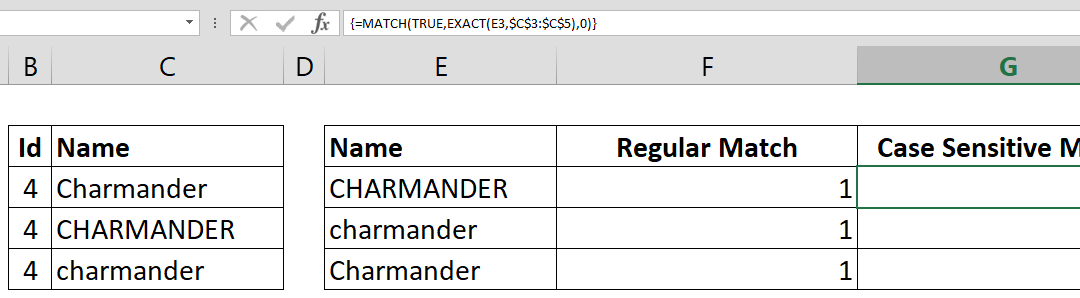## How to speed up lookup formulas

Lookup functions are extremely useful when working with large data sets. Although they almost always work instantaneously with small data, larger data sets is when things can get rough in terms of performance, as large operations can require more computational...## How to find closest match

This article shows How to find closest match by using INDEX, MATCH, ABS and MIN functions. Excel’s array formula ability to evaluate formula for each cells in an array is key factor in this case. Syntax { =INDEX( return array,      MATCH(         MIN(ABS( search...## How to get Excel cell address of a lookup result

This article shows you how to get Excel cell address of a lookup result by using the CELL and INDEX functions. Syntax =CELL( “address”, INDEX( data, MATCH( lookup value for row, lookup range for row, 0 ), column index ) ) Steps Start with the =CELL(...## How to make case sensitive match

If your data is case sensitive, you may have issues finding correct matches with lookup functions. This How to make case sensitive match article shows you how to combine MATCH and EXACT functions to achieve case sensitive matching. Syntax { =MATCH(TRUE, EXACT(search...